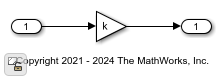# Reference a Subsystem File in a Model

You can save a subsystem in an SLX or MDL file and reference it from a model. Such a modeling pattern helps re-usability. Consider the `slexSSRef_model` model. This model contains two subsystems referencing the same subsystem file - `TimesK.slx`. The `TimesK.slx` subsystem contains Inport, Gain, and Outport blocks and is masked using Model Mask. An Input for Gain parameter is added to the mask to pass value using the variable `k`. This model is configured to demonstrate these capabilities of a referenced subsystem.

### Instance Specific Parameterization

You can specify different parameter values for each instance of a referenced subsystem. For example, here the input value (k) for the Input for Gain parameter from Subsystem1 and Subsystem2 are `5` and `10` respectively.

### Instance Specific Debugging

In this example, you have two instances of subsystem, referencing the saved subsystem file, `TimesK`. Each instance is driven by its own set of inputs in the top model. When you need to debug a referenced subsystem, you can dive into each instance and look for errors and debug them.

While referencing subsystems, the top model can drive multiple dimensions. In this model, the Subsystem1 instance is driven by an one-dimensional signal and the Subsystem2 instance is driven by a four-dimensional signal. In both instances, you can see that the referenced subsystems adapt to have one dimension and four dimensions respectively in the output.